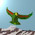## Thursday, January 5, 2012

### Dark Matter Update

Let us recall the basic dark matter fractions in the quark gluon plasma. That's $3 / 4 \pi$ for the dark matter fraction and $9/ 4 \pi$ for the (so called) dark energy, with the baryonic fraction $\Omega_{b} = 1 - 3/ \pi$, as confirmed by WMAP.

In the information theory, we need two kinds of dimension. First, path bases define an integral dimension, $27$ for the Jordan algebra. But complex matrix entries tend to be characters for finite fields, so that the root of unity corresponding to a phase $2 \pi /27$ would specify the cardinality of a field with $27$ elements. These two dimension types should be related by the mirror physics that explains the dark matter fractions.

A dark matter ansatz: the total dark sector density fraction $\Omega_{d}$, as a fraction of the character dimension for $27$ paths, equals the observable universal fraction of the integral dimension. In other words, we would like to write

$\frac{3}{\pi} \frac{2 \pi}{27} = \frac{2}{9}$

so that $\Omega_{d} = 3/ \pi$. Now observe that we can split the dark sector into quark and lepton components. The leptonic dark matter fraction $3/ 4 \pi$ now corresponds to the fraction $1/18$, a single quark path from the quark set. Similarly, the dark energy fraction $9/ 4 \pi$ corresponds to the fraction $1/6$, a single lepton path. The total $3/ \pi$ is then the only solution obeying this quark lepton complementarity.

In the quark gluon plasma, the minimal viscosity to entropy ratio is $1/ 4 \pi$. As a character dimension fraction, $1/ 4 \pi$ corresponds to $1/54$, a single basis path in the full bioctonion algebra.

1.If you really want to drive yourself mad, try to bring into the mix the fact that the Sun rotates through about 2π/27 radians in one Earth day...!

2.Wow, Mitchell, it does? But why is that crazy? After all, the sun-earth system is one of the most fundamental clocks for a human observer, no?

3.Hmmm. I guess the crazy aspect comes from the existence of other planets in the solar system, with varying length days. But Earth is rather unique, no? Was humanity not given dominion over the solar system?

4.I see it like this: The solar system contains many resonances, and therefore to some extent it really does manifest algebraic order (e.g. Bode's law). Meanwhile, in this post you are seeking to explain a "numerology" from a quite different part of astrophysics, in terms of deep algebraic relations. So it could be that there is some overlap in the algebra but not in the physics - that is, the fact that (e.g.) 27 = 3^3 might have some significance in both cases, but it would be a different significance, with no overlap in physical context. The other option is that there is an overlap, and I'm sure that if a person were sufficiently imaginative, they could get somewhere with this (e.g. by starting from Louise's thesis about black holes in planets). I am torn between wanting to focus just on what you wrote about in the post, and wanting to try out the wild idea of a genuine link. Fortunately, it's not really a dilemma, the two trains of thought can run in parallel... But for further comments on this post, maybe it would be desirable just to stick to the original idea.

5.I agree, Mitchell. I feel we will progress further, at this stage, if we focus on the fundamental algebraic questions, and hence dark matter properties and so on. But yes, there must be some spectacular predictions for solar system physics here (and other local human-scale physics) based on the new cosmology.

So at present I am thinking more about the consequences of neutrino/nuclear physics than the Riofrio Earth black hole, which must somehow be the source of period coincidences in the solar system. Perhaps all the solar system planets started with a basic period, with respect to the sun, but as the black hole cores died off the periods were modified, except for the Earth.

Recall that we did look at 'Bode's law' a while back, and one can really see the quantization in the other known star systems.

6.Thanks again for the mention!

7.Kea,

I just saw Ulla's lost thread posts and found quite a discussion and debate I am still reading. Also I commented on Pitkanen's post today on the super-luminal neutrinos and posted this in the comment section:

Matti and all,

You may have noticed that in this discussion of superluminal neutrinos I used the special word teleomnic or tachyonic-like to describe such apparent phenomena.

The general theories, the supersymmetry of myself and Kea still stands, and always did.

The PeSla

BTW I have not just blindly supported your ideas but given them an objective evaluation.

Mitchell, if you value the opinions of armatures I am also one to interview as you suggested maybe better in e-mail.

The PeSla

8.Hi The Pe Sla and Louise. Louise, I don't believe I ever saw a paper by you that focuses on the $1$ to $3$ split for DM, although we have discussed it for years now. Perhaps it is in the literature somewhere?

Note: Only a member of this blog may post a comment.